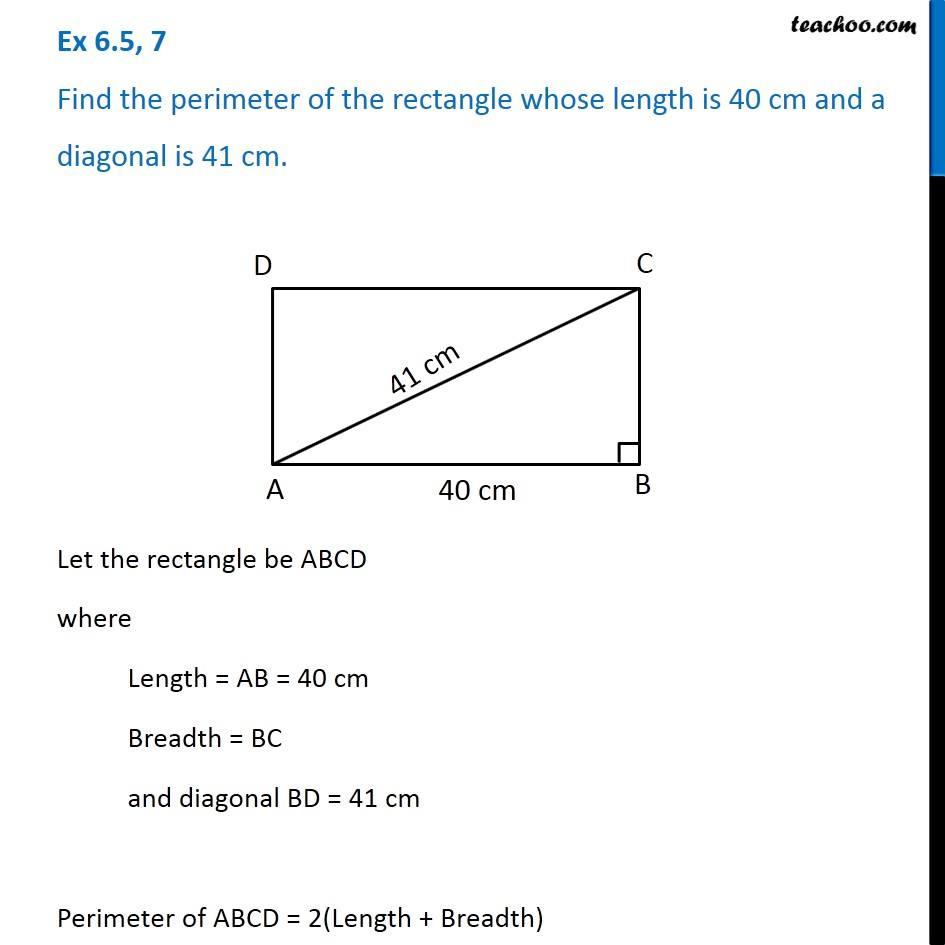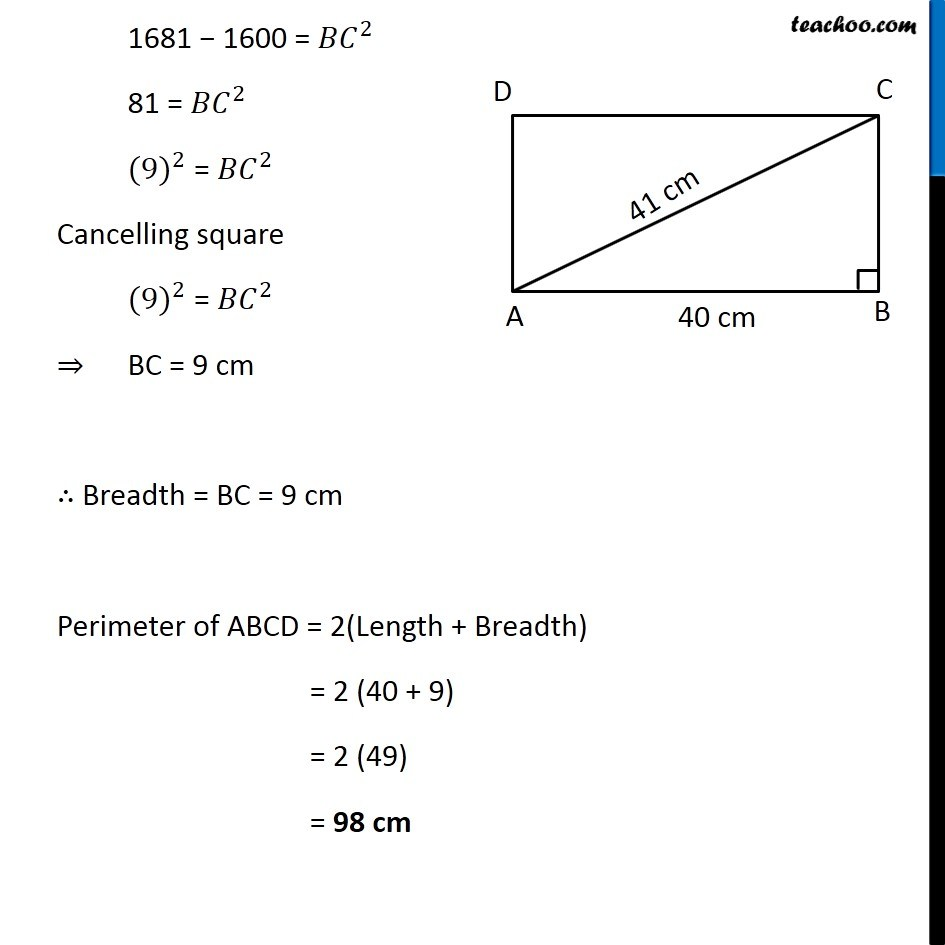Ex 6.5

Chapter 6 Class 7 Triangle and its Properties
Serial order wiseLearn in your speed, with individual attention - Teachoo Maths 1-on-1 Class

### Transcript

Ex 6.5, 7 Find the perimeter of the rectangle whose length is 40 cm and a diagonal is 41 cm. Let the rectangle be ABCD where Length = AB = 40 cm Breadth = BC and diagonal BD = 41 cm Perimeter of ABCD = 2(Length + Breadth) Let’s find breadth BC In rectangle, all angles are 80° ∴ ∠A = ∠B = ∠C = ∠D 80° Therefore, In right triangle Δ ABC By Pythagoras Theorem, 〖𝐴𝐶〗^2 = 〖𝐵𝐶〗^2 + 〖𝐴𝐵〗^2 〖(41)〗^2 = 〖𝐵𝐶〗^2 + 〖(40)〗^2 1681 = 〖𝐵𝐶〗^2 + 1600 1681 − 1600 = 〖𝐵𝐶〗^2 1681 − 1600 = 〖𝐵𝐶〗^2 81 = 〖𝐵𝐶〗^2 〖(9)〗^2 = 〖𝐵𝐶〗^2 Cancelling square 〖(9)〗^2 = 〖𝐵𝐶〗^2 ⇒ BC = 9 cm ∴ Breadth = BC = 9 cm Perimeter of ABCD = 2(Length + Breadth) = 2 (40 + 9) = 2 (49) = 98 cm